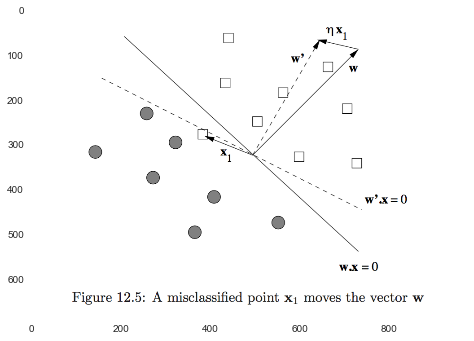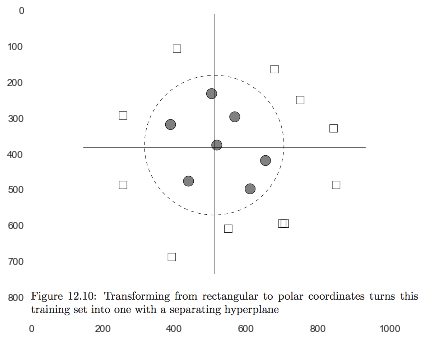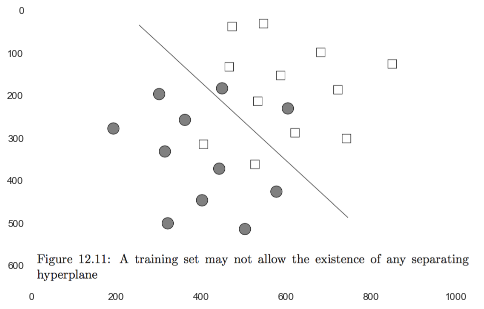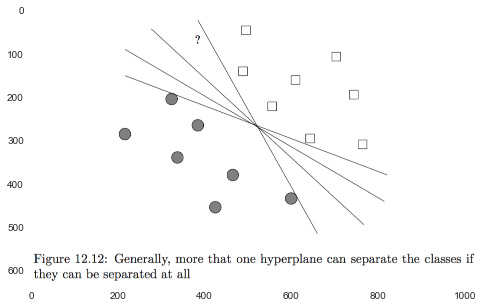In :
# %load ../../preconfig.py
%matplotlib inline
import matplotlib.pyplot as plt
import seaborn as sns
sns.set(color_codes=True)
plt.rcParams['axes.grid'] = False

#import numpy as np
#import pandas as pd

#import sklearn

#import itertools

import logging
logger = logging.getLogger()

def show_image(filename, figsize=None, res_dir=True):
if figsize:
plt.figure(figsize=figsize)

if res_dir:
filename = './res/{}'.format(filename)



# 12 Large-Scale Machine Learning¶

All algorithms for analysis of data are designed to produce a useful summary of the data, from which decisions are made.

"machine learning" not only summarize our data; they are perceived as learning a model or classifier from the data, and thus discover something about data that will be seen in the future.

### 12.1 The Machine-Learning Model¶

Training sets:

• feature vector: $x$
• label: $y$
• real number, regression
• boolean value, binary classification
• finite set, multiclass classification
• infinite set, eg: parse tree

#### 12.1.3 Approaches to Machine Learning¶

1. Decision trees suitable for binary and multiclass classification small features

2. Perceptrons $\sum w x \geq \theta$ binary classification, very large features

3. Neural nets binary or multiclass classification

4. Instance-based learning use the entire training set to represent the function $f$. eg: k-nearest-neighbor

5. Support-vector machines accureate on unseen data

#### 12.1.4 Machine-Learning Architecture¶

1. Training and Testing

2. Batch Versus On-Line Learning on-line:

• very large training sets
• adapt to changes as times goes
• active learning
3. Feature Selection

4. Creating a Training Set

In :
#exercise


### 12.2 Perceptrons¶

The output of the perceptron is:

• $+1$, if $w x > 0$
• $-1$, if $w x < 0$
• wrong, if $w x = 0$

The weight vector $w$ defines a hyperplane of dimension $d-1$ where $w x = 0$.

A perceptron classifier works only for data that is linearly separable.

#### 12.2.1 Training a Perceptron with Zero Threshold¶

1. Initialize $w = 0$.

2. Pick a learning-rate parameter $\eta > 0$.

3. Consider each training example $t = (x, y)$ in turn.

1. $y' = w x$.
• if $y'$ and $y$ have the same sign, then do nothing.
• otherwise, replace $w$ by $w + \eta y x$.
That is, adjust $w$ slightly in the direction of $x$.
In :
show_image('fig12_5.png')#### 12.2.2 Convergence of Perceptrons¶

data point are not linearly separable $\to$ loop infinitely.

some common tests for termination:

1. Terminate after a fixed number of rounds.

2. Terminate when the number of misclassified training points stops changing.

3. Terminate when the number of errors on the test set stops changing.

Another technique that will aid convergence is to lower the traing rate as the number of rounds increases. eg: $\eta = \eta / (1 + ct)$.

#### 12.2.3 The Winnow Algorithm¶

Winnow assumes that the feature vectors consist of 0's and 1's, and the labels are $+1$ or $-1$. And Winnow produce only positive weithts $w$.

idea: there is a positive threshold $\theta$.

1. if $w x > \theta$ and $y = +1$, or $w x \theta$ and $y = -1$, then do nothing.

2. if $w x \leq \theta$, but $y = +1$, then the weights for the components where $x$ has 1 are too low as a group, increase them by a factor, say 2.

3. if $w x \geq \theta$, but $y = -1$, then the weights for the components where $x$ has 1 are too high as a group, decreae them by a factor, say 2.

#### 12.2.4 Allowing the Threshold to Vary¶

At the cost of adding another dimension to the feature vectors, we can treate $\theta$ as one of the components of $w$.

1. $w' = [w \theta]$.

2. $x' = [x -1]$.

3. We allow a $-1$ in $x'$ for $\theta$ if we treat it in the manner opposite to the way we treat components that are 1.

one VS all

#### 12.2.6 Transforming the Training Set¶

turn to linear separable set.

In :
show_image('fig12_10.png')#### 12.2.7 Problems With Perceptrons¶

1. The biggest problem is that sometimes the data is inherently not separable by a hyperplane.

transforming $\to$ overfitting.

2. many different hyperplanes that will separate the points.

In :
show_image('fig12_11.png')In :
show_image('fig12_12.png')#### 12.2.8 Parallel Implementation of Perceptrons¶

training examples are used with the same $w$.

map: calculate $w$ independently.

reduce: sum all $w$.

In :
#Exercise


### 12.5 Comparison of Learning Methods¶

In [ ]: